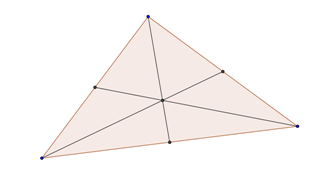# CentroidAllPages RecentChanges Links to this page Edit this page Search Entry portal Advice For New UsersThe centroid of a triangle is located at the intersection of the medians of the triangle. Depending on context, this point is also known as the centre of mass or centre of gravity of the triangle. If you make a model of the triangle out of some flat, rigid material, the centroid is the point at which you can balance it on a point. In particular, any line through the centroid divides the triangle into two pieces of equal area.

Taking that last sentence as the definition of "centroid" for any two-dimensional shape, every such shape has a centroid. (I am handwaving a bit about the definition of "shape" here.) Here is a very rough sketch of a proof. For each point in the shape, consider all lines through that point and look at the biggest difference you can get between the area of the shape on the two sides of the line. Find a point that minimizes this number. The number there must be nonzero, because otherwise you can decrease it by moving perpendicular to a line through that point that gives the maximum area difference.

Shapes in more than two dimensions also have centroids. (In three dimensions: every plane through the centroid divides the shape into two pieces of equal volume. In more than three dimensions: every hyperplane through the centroid divides the shape into two pieces of equal hypervolume.)